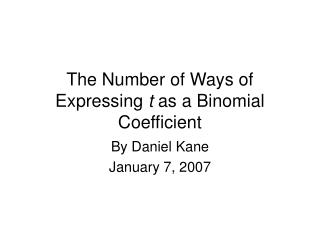# The Number of Ways of Expressing t as a Binomial Coefficient - PowerPoint PPT PresentationDownload PresentationThe Number of Ways of Expressing t as a Binomial Coefficient

The Number of Ways of Expressing t as a Binomial Coefficient
Download Presentation## The Number of Ways of Expressing t as a Binomial Coefficient

- - - - - - - - - - - - - - - - - - - - - - - - - - - E N D - - - - - - - - - - - - - - - - - - - - - - - - - - -
##### Presentation Transcript

1. The Number of Ways of Expressing t as a Binomial Coefficient By Daniel Kane January 7, 2007

2. An Interesting Note: 10 is both a triangular number and a tetrahedral number.

3. Why does this hold? Is it equal to any other binomial coefficient?

4. Trying to produce solutions of the form Are there any non-trivial relations? Yields a Pell Equation with Solutions

5. Define Stating the Problem More Precisely • Studied by Singmaster in  (1971) • N(3003) = 8 • N(t) ¸ 6 for infinitely many t • Conj: N(t) = O(1)

6. What are Reasonable Bounds? • Singmaster showed in  that • Consider n > 2m (we do this from now on)

7. An improvement • In  Erdös et al. proved that • Prime in (n-n5/8, n) for n >> 1. • Split into cases based on n > (log t)6/5

8. For n > (log t)6/5 • Use Approximation

9. For n < (log t)6/5 • Approximation yields m > n5/8 • 9 prime, P, s.t. n-m < P < n • P divides t • Pick largest N, all others satisfy P < n < N • At most M solutions • M = O(N5/8) = O((log t)3/4) • Using the strongest conjectures on gaps between primes could give

10. Another way to handle this case • Consider all solutions • Order so n1 > n2 > … > nk m1 < m2 < … < mk, • As n decreases and m increases, the effect of changing n decreases and the effect of changing m increases. • (ni-ni+1)/(mi+1-mi) increasing. • These fractions are distinct

11. Another way (continued) • Differences of (ni , mi) are distinct • Only O(s2) have n, m < s • Total Change in n-m > ck3/2 • k3/2 = O((log t)6/5) • k = O ((log t)4/5).

12. What did we do? • n convex function of m • Lattice points on graph of ANY convex function • Idea: Consider higher order derivatives.

13. Problems to Overcome • How do we use the derivative data? • How do we obtain the derivative data?

14. Using Data, a Lemma Lemma If f and g are Cn-functions so that f(x) = g(x) at x1 < x2 < … < xn+1, then f(n)(y) = g(n)(y) for some y2 (x1 , xn+1) • Generalization of Rolle’s Theorem • Proof by induction & Rolle’s Theorem

15. y f(n)(x) = g(n)(x) f(2)(x) = g(2)(x) f(1)(x) = g(1)(x) f(0)(x) = g(0)(x) x1 x2 x3 xn-1 xn xn+1 Using Data, Proof of Lemma

16. Using Derivative Data (Continued) • Consider function f, f(mi) = ni • g polynomial interpolation of f at m1, m2, …, mk+1 • g(k) constant • f(k) is this constant at some point

17. Using Derivative Data (cont.)

18. Using Derivative Data (cont.) • kth derivate of f small but non-zero • Fit polynomial to k+1 points separated by S • ) kth derivative over k! either 0 or more than S-k(k+1)/2.

19. Derivative Data • Define • Make smooth using  • Estimate with Sterling’s approximation

20. Estimating Derivatives • Main Term: Derivatives of [ log t + (z+1) ] / z and take exp of power series • (z - 1) / 2 is easy • Error term: Cauchy Integral Formula

21. A Useful Parameter

22. Split into Cases •  < 1.15 • 1.15 <  < log log t/(24 log log log t) • log log t/(24 log log log t)<  <(log log t)4 • (log log t)4 < 

23. Case 1:  < 1.15 • Already covered • O((log t)3/4) solutions

24. Case 2: 1.15 <  < log log t/(24 log log log t) • Set k = (log log t)/(12 log log log t) • Technical conditions satisfied • k+1 adjacent solutions mi of separation S

25. Case 3: log log t/(24 log log log t)<  <(log log t)4 We need a slightly better analysis to bound LCM over all sequences of k distinct ri 0, |ri| < S

26. Bounding B • Count multiples of each prime • pn divides at most min(k , 2S/pn) ri’s • Use Prime Number Theorem

27. Using Bound • k = 2 • Technical Conditions Satisfied

28. Case 4: (log log t)4 < 

29. Conclusions • Know where to look to tighten this bound • Can use technique for other problems

30. References  D. Kane, On the Number of Representations of t as a Binomial Coefficient, Integers: Electronic Journal of Combinatorial Number Theory,4, (2004), #A07, pp. 1-10.  D. Singmaster, How often Does an Integer Occur as a Binomial Coefficient?, American Mathematical Monthly, 78, (1971) 385-386  H. L. Abbott, P. Erdös and D. Hanson, On the number of times an integer occurs as a binomial coefficient, American Mathematical Monthly, 81, (1974) 256-261.